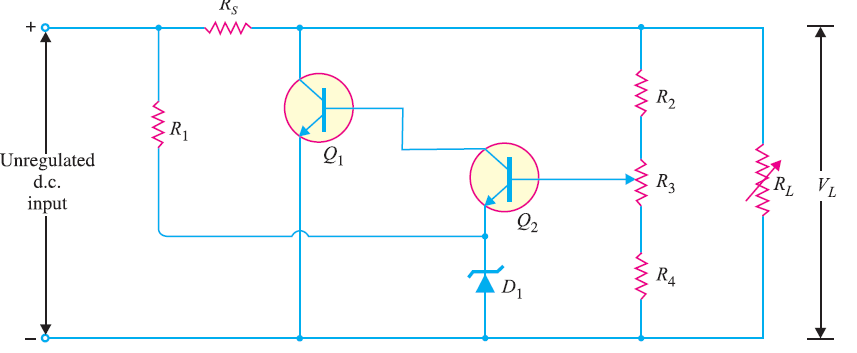# Shunt Feedback Voltage Regulator

## Shunt Feedback Voltage Regulator

You can see the circuit diagram of a shunt feedback voltage regulator below.This circuit uses an error detector (Q2) to control the conduction of a shunt transistor (Q1). Note that transistor Q1 is in parallel with the load, it is called shunt transistor and hence the name of the regulator.The error detector (Q2) receives two inputs : a reference voltage and a sample voltage. The sample circuit is a simple voltage divider circuit (R2 – R3 – R4) and derives the sample voltage from the regulated output voltage. The reference circuit is made up of zener D1 and R1 and derives the reference voltage from the unregulated d.c. input voltage. The outputs from the sample and reference circuits are applied to the error detector (Q2). The output from Q2 is used to control the conduction of the shunt transistor Q1. Since Q1 (shunt transistor) is in parallel with load RL, the change in the current conduction through Q1 can control the load voltage.

### Working of Shunt Feedback Voltage Regulator

In a shunt feedback voltage regulator, the outputs from the sample and reference circuits are applied to the error detector/amplifier Q2. The output from Q2 controls the conduction current through the shunt transistor Q1 to maintain the constant load voltage VL.

• Suppose the load voltage VL decreases due to any reason. This decrease causes the base voltage of Q2 [VB (Q2)] to decrease. Since emitter voltage of Q2 [VE (Q2)] is set to a fixed value (VZ) by the zener diode, VBE (Q2) decreases when VB (Q2) decreases. This decreases base current IB (Q1) through the base of transistor Q1. This in turn causes IC1 to decrease; thus increasing VC (Q1). Since VL = VC (Q1), the increase in VC (Q1) offsets the initial decrease in VL.
• Suppose the load voltage increases due to any reason. This increases the conduction through Q2, causing an increase in IB (Q1). The increase in IB (Q1) causes the shunt transistor’s conduction to increase, decreasing VC (Q1). The decrease in VC (Q1) offsets the initial increase in VL.

You Might Like The Following Articles